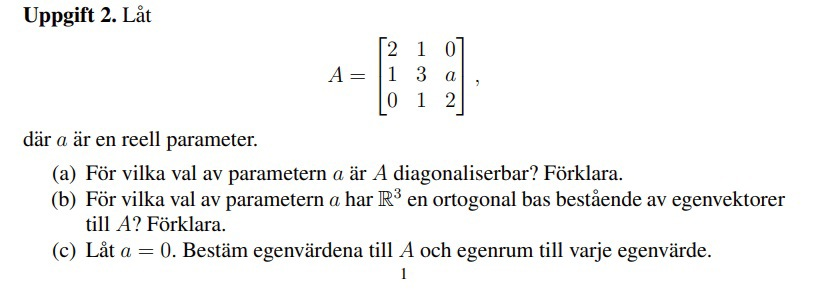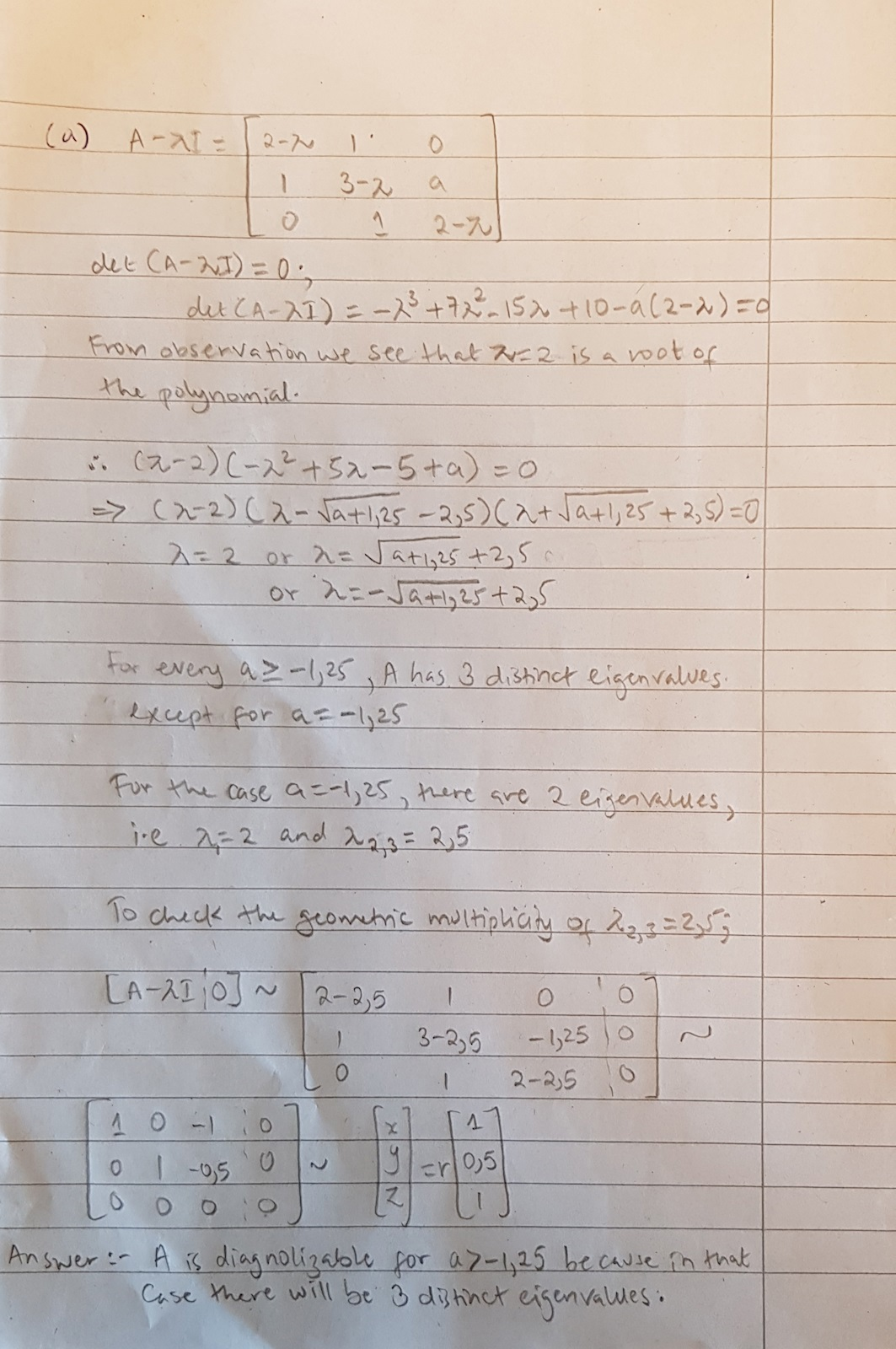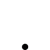21 svar
112 visningar
Nox_M är nöjd med hjälpen

# Linjär Algebra(a)När jag försöker lösa 'det(A-λI)=0' så får jag '-x³+7x²+ax-15x+10-2a=0'. Hur kan jag lösa den när jag har fler okända variabler?

Finns det något annat sätt att svara på (a) än att först hitta eigenvärde och eigenvektorer?

(Ps: I am not sure if my Swedish is correct so if my question is confusing, please ask me!

To the mods: is it allowed to write in English instead of Swedish?)

Although you could attempt to calculate the eigenvalues/vectors and check the condition A=PDP^(-1), it is not at all necessary.

A matrix is diagonalizable if and only if for each eigenvalue the dimension of the eigenspace is equal to the multiplicity of the eigenvalue.

That is, suppose we have a 2x2 square matrix. It should be obvious that if n denotes rows, then n=2. If we can find two (n distinct) distinct eigenvalues for this given 2x2 matrix then we can conclude that it is indeed diagonalizable without having to show that A=PDP^(-1).

As far as I know, writing your thread in English is not per se breaking the rules but keep in mind you'll be far less likely to recieve help.

My main issue is how to find the eigenvalues. If I use det(A-λI)=0 then I get an equation that has too many unknowns. Is there an alternative method I can use to find the eigenvalues?

There is a lucky root to your characteristic polynomial, x=2. You can then factor the polynomial using polynomial division.

Once you have done that you will be able to see that there are 3 distinct eigenvalues in all cases but one. With 3 distinct eigenvalues, diagonalizability follows from gm <= am with a minimum of 1 for gm. In the special case you can simply substitute that value for a into your original matrix and manually evaluate the situation.

I have ignored complex solutions here.

So I factored the polynomial and got:

(λ-2)(-λ²+5λ-5+a)=0

I don't know where to go from here. How do I factor (-λ²+5λ-5+a)?

complete the square

I did that and I got

λ = a + 3,75

So does that mean that A is diagonalizable for all real number values of a? is -3,75 included?

write out the polynomial with only linear factors

I completed the square but I made a wrong assumption along the way

-λ² + 5λ - 5 + a=0

λ² - 5λ = a - 5

λ² - 5λ + 6,25 = a - 5 + 6,25

(λ - 2,5)(λ - 2,5) = a + 1,25

(λ - 2,5)² = a + 1,25

λ - 2,5 = ±√(a + 1,25)

λ  = ±√(a + 1,25) + 2,5

Have I done it correctly? and if so can I assume that A is diagonalizable for all numbers when a ≥1,25?

it looks correct,

now write the polynomial like this -(x-a1)(x-a2)(x-a3)

(λ  + √(a + 1,25) - 2,5)(λ - √(a + 1,25) - 2,5)(λ - 2)= 0

So, I see that for any choice of a≥1,25 there are 3 distinct eigenvalues except in the case when a=1,25, there are two eigenvalues, λ=2 and λ=2,5 (multiplicity 2)

Am I on the right track?

yes now u must evaluate the case a=1.25 manually by inserting it into your original matrix

Why do I have the evaluate the special case of a=1,25 manually?

Can I not assume that since a=1,25 gives me two eigenvalues one of which has multiplicity 2, it automatically satisfies the condition that there are 3 eigenvalues for the 3 x 3 matrix?

u dont know what the geometirc multiplicity of the a=1.25 is. you need to determine which vectors are associated with the a=1.25 case

I will put everything in writing and post it and if you have time later you can give me feedback.

Thank you very much for all the help so far! : )I skipped the calculation steps (polynomial division, completing the square, row reduction) so that the information on the page is readable. Is my answer correct?

I get the impression that you need to have good look in your textbook reguarding diagonalization and geometric and algebraic multiplicity.

For the case of a=-1.25 we have established that there are 2 eigenvalues, x=2 and 2.5. You still have to investigate what vectors belong to the x=2 eigenvalue.

Yeah, my knowledge of linear algebra is very superficial. I need to do a whole lot of revision.

There is one eigenvector that belongs to λ=2

I automatically assumed that since λ=2 has multiplicity 1, it could only give me one eigenvector. Was my assumption wrong?

Now that I found that there are only 2 eigenvectors when a=-1.25, does that change my answer?

Thank you for being so patient with me : )